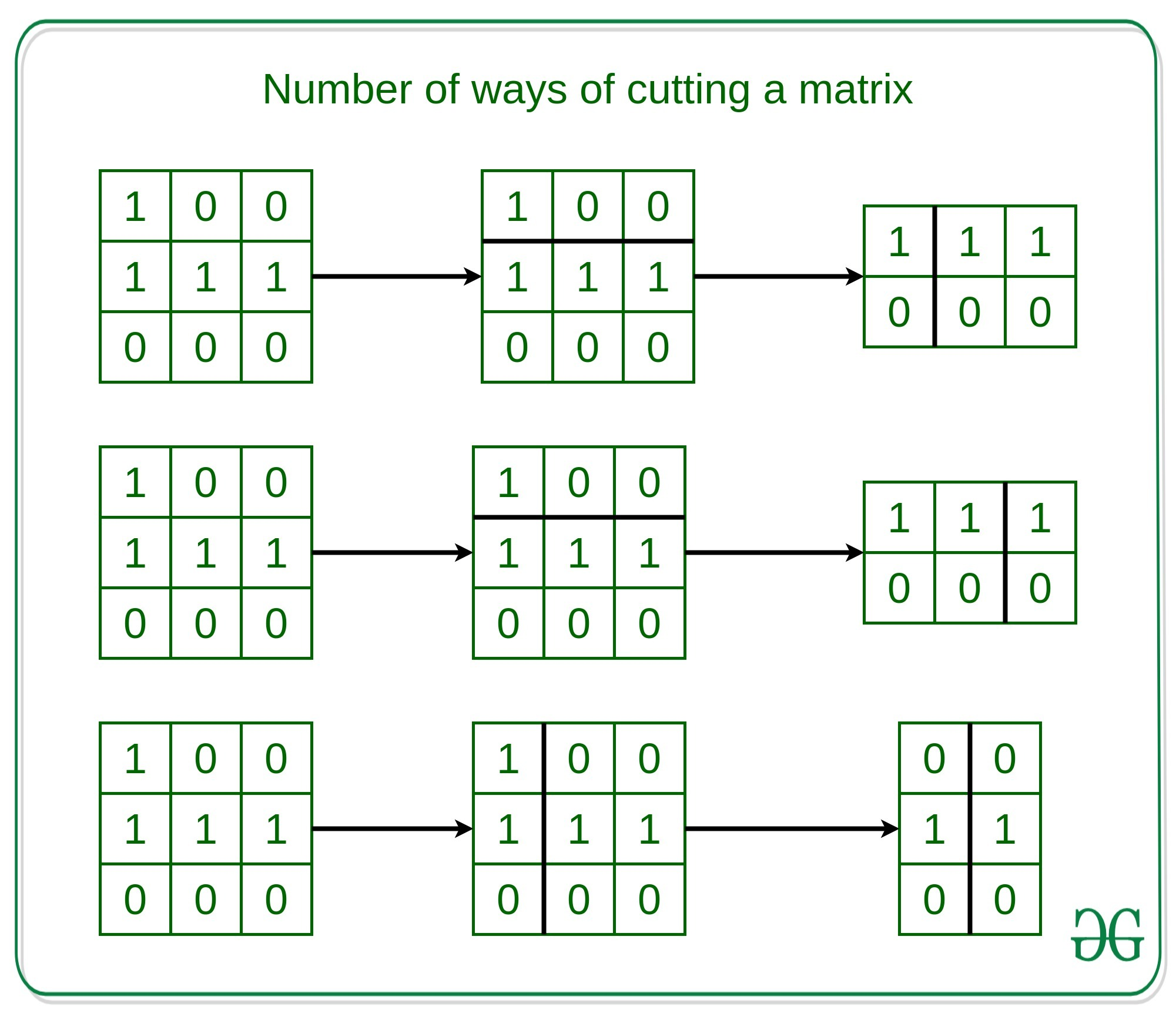# Number of ways of cutting a Matrix such that atleast one cell is filled in each part

Given an integer K and a matrix mat[][] containing 1 and 0, where 1 denotes the cell is filled and 0 denotes an empty cell. The task is to find the number of ways to cut the matrix into K parts using K-1 cuts such that each part of the matrix contains atleast one filled cell.

For each cut, there must be a direction either horizontal or vertical. Then you choose a cut position at the cell boundary and cut the matrix into two parts. If you cut the matrix vertically, the left part of the matrix will not be used again for further process. If you cut the matrix horizontally, the upper part of the matrix will not be used again.

Examples:

Input: mat[][] = {{1, 0, 0}, {1, 1, 1}, {0, 0, 0}}, K = 3
Output: 3
Explantion:Input: matrix = {{1, 0, 0}, {1, 1, 0}, {0, 0, 0}}, K = 3
Output: 1

Approach: The idea is to use Dynamic Programming to compute the number of ways of cutting a matrix with atleast one filled cell.

• Compute the prefix sum of the matrix such that we can compute a particular portion of the matrix contains a filled cell or not in O(1) time.
• Define a dp table to store the number of ways to cut the pizza in K parts, where dp[k][r] denotes the number of ways to cut the matrix into K parts from top left to Rth row and Cth Column.
• Finally, iterate over for every possible matrix and check that if the matrix can be cut into the two parts upto that index and both part is valid or not.

Below is the implementation of the above approach:

## Python

 `# Python implementation to find the  ` `# number of ways to cut the matrix  ` `# into the K parts such that each ` `# part have atleast one filled cell ` ` `  `# Function  to find the  ` `# number of ways to cut the matrix  ` `# into the K parts such that each ` `# part have atleast one filled cell ` `def` `ways(arr, k): ` `    ``R ``=` `len``(arr) ` `    ``C ``=` `len``(arr[``0``]) ` `    ``K ``=` `k ` `    ``preSum ``=` `[[``0` `for` `_ ``in` `range``(C)]\ ` `                 ``for` `_ ``in` `range``(R)] ` `                  `  `    ``# Loop to find prefix sum of the  ` `    ``# given matrix ` `    ``for` `r ``in` `range``(R``-``1``, ``-``1``, ``-``1``): ` `        ``for` `c ``in` `range``(C``-``1``, ``-``1``, ``-``1``): ` `            ``preSum[r] ``=` `arr[r] ` `             `  `            ``if` `r ``+` `1` `< R: ` `                ``preSum[r] ``+``=` `preSum[r ``+` `1``] ` `            ``if` `c ``+` `1` `< C: ` `                ``preSum[r] ``+``=` `preSum[r] ` `                 `  `            ``if` `r ``+` `1` `< R ``and` `c ``+` `1` `< C: ` `                ``preSum[r] ``-``=` `preSum[r ``+` `1``] ` `     `  `    ``# dp(r, c, 1) = 1  ` `    ``# if preSum[r] else 0 ` `    ``dp ``=` `[[[``0` `for` `_ ``in` `range``(C)]\ ` `              ``for` `_ ``in` `range``(R)]\ ` `              ``for` `_ ``in` `range``(K ``+` `1``)] ` `               `  `    ``# Loop to iterate over the dp  ` `    ``# table of the given matrix ` `    ``for` `k ``in` `range``(``1``, K ``+` `1``): ` `        ``for` `r ``in` `range``(R``-``1``, ``-``1``, ``-``1``): ` `            ``for` `c ``in` `range``(C``-``1``, ``-``1``, ``-``1``): ` `                ``if` `k ``=``=` `1``: ` `                    ``dp[k][r] ``=` `1` `\ ` `                        ``if` `preSum[r] > ``0``\ ` `                        ``else` `0` `                ``else``: ` `                    ``dp[k][r] ``=` `0` `                    ``for` `r1 ``in` `range``(r ``+` `1``, R): ` `                         `  `                        ``# Check if can cut horizontally ` `                        ``# at r1, at least one apple in  ` `                        ``# matrix (r, c) -> r1, C-1 ` `                        ``if` `preSum[r] ``-` `preSum[r1] > ``0``: ` `                            ``dp[k][r] ``+``=` `dp[k``-``1``][r1] ` `                    ``for` `c1 ``in` `range``(c ``+` `1``, C): ` `                         `  `                        ``# Check if we can cut vertically  ` `                        ``# at c1, at least one apple in  ` `                        ``# matrix (r, c) -> R-1, c1 ` `                        ``if` `preSum[r] ``-` `preSum[r][c1] > ``0``: ` `                            ``dp[k][r] ``+``=` `dp[k``-``1``][r][c1] ` `    ``return` `dp[K][``0``][``0``] ` `     `  `# Driver Code ` `if` `__name__ ``=``=` `"__main__"``: ` `    ``arr ``=` `[[``1``, ``0``, ``0``], [``1``, ``1``, ``1``], [``0``, ``0``, ``0``]] ` `    ``k ``=` `3` `     `  `    ``# Function Call ` `    ``print``(ways(arr, k)) `

Output:

```3
```

Don’t stop now and take your learning to the next level. Learn all the important concepts of Data Structures and Algorithms with the help of the most trusted course: DSA Self Paced. Become industry ready at a student-friendly price.

My Personal Notes arrow_drop_upCheck out this Author's contributed articles.

If you like GeeksforGeeks and would like to contribute, you can also write an article using contribute.geeksforgeeks.org or mail your article to contribute@geeksforgeeks.org. See your article appearing on the GeeksforGeeks main page and help other Geeks.

Please Improve this article if you find anything incorrect by clicking on the "Improve Article" button below.